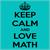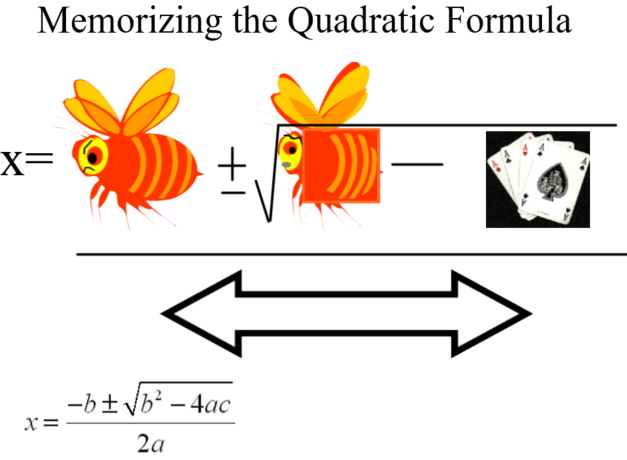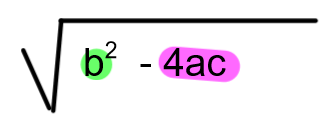# My NCTM (All Members)

last person joined: 2 days ago

Follow#### Lane Walker11-16-2022 22:06#### Ancy Mariam Joseph11-25-2022 22:02#### Edric Cane11-28-2022 22:27#### Tayah Rendina12-12-2022 13:28#### Lane Walker12-12-2022 14:32#### Edward Thome12-13-2022 10:21#### Henri Picciotto12-13-2022 11:51#### Edric Cane12-13-2022 21:40#### David Srebnick12-14-2022 04:42• #### 1.  Visualizing the Quadratic Formula

Posted 11-16-2022 22:06
Great teachers don't miss opportunities to bring in all the senses to make something "stick."  The proliferation of Quadratic Formula songs on Youtube bears witness of that.  But when students confuse b and c because they rhyme, a song doesn't necessarily cover all the bases for memory.  Here's how I cover the visual component:------------------------------
Lane Walker, Ed.D
High School Math Teacher
Fuquay-Varina, NC
------------------------------

• #### 2.  RE: Visualizing the Quadratic Formula

Posted 11-25-2022 22:02
Thank you Lane, for sharing this.

------------------------------
Ancy Mariam Joseph
Engineer | Educator for 8 years | Math Department Lead
Single Subject Math Credential (CA)
Graduate Student @Notre Dame School of Education
San Francisco Bay Area
CA
------------------------------

• #### 3.  RE: Visualizing the Quadratic Formula

Posted 11-28-2022 22:27

However students memorize the formula, I like them to also think of the version where dividing by '2a' appears twice: -b/2a + or - (Sq rt of (b2 – 4ac))/2a. This gives meaning to the formula: the first term (-b/2a) is the x-value of the axis of the parabola; + or – a positive second term is the distance on either side of that axis to the parabola itself.

Given an example of a quadratic equation, y = ax2 + bx + c, I want students to use it to visualize the parabola:

With x = 0, y = c:  'c' is the parabola's y-intercept.

Y = bx + c is a line through c that is easy to visualize ('c' is y-intercept, slope is 'b'). It is tangent to the parabola at c.

'ax2' is the curving factor that applies to the y = bx + c line: it makes the line curb back on itself changing it into the parabola.

If we cut out a paper parabola for a = 1, for instance, it is easy to position it on a graph tangent to line y = bx + c. This immediately tells us where the roots would be (if there are real roots). It immediately shows that, if 'a' and 'c' are positive, any real root must both be negative. If 'a' is positive and 'b' negative, we immediately know that both roots, if real, must be positive. Just by looking at the equation, students can learn to visualize the graph of the parabola and immediately see many of its essential characteristics.

------------------------------
Edric Cane
Math teacher, retired
Author,
Teaching to Intuition: Mathematics.
Workbook: Making Friends with Numbers: Let learning Multiplication Facts teach you Math.
edriccane@aol.com
(916) 973-8569.
Carmichael, CA 95608
------------------------------

• #### 4.  RE: Visualizing the Quadratic Formula

Posted 12-12-2022 13:28
Hi Lane and Edric,

I think this visual is fun for students to see and could help with memorization. I am a first-year algebra 1 teacher getting ready to teach quadratic formula.

While this is helpful in making it stick, I do like what Edric discusses in deriving the formula from the standard form of a quadratic equation. I think I will definitely have student discover these connections before showing them this visual. For our state testing, students will be given the formula on their reference sheet. I want them to understand WHY it works and how to reason through finding solutions.

Thank you for sharing!

------------------------------
Tayah Rendina
Salisbury MD
------------------------------

• #### 5.  RE: Visualizing the Quadratic Formula

Posted 12-12-2022 14:32
I absolutely agree with not having students memorize the quadratic formula without seeing how it is derived.  Since so many math teachers struggle to get through the proof perfectly, I have not yet had a class that I expected to re-generate the proof; but we work through it in a whole-class discussion.

As a contributor to Nix The Tricks and a huge fan of "13 Rules that Expire," I don't recommend even teaching things like "cross products," so thank you for bringing that up!

------------------------------
Lane Walker, Ed.D
High School Math Teacher
Fuquay-Varina, NC
------------------------------

• #### 6.  RE: Visualizing the Quadratic Formula

Posted 12-13-2022 10:21
Thank you for saying this, and for the reference to Nix The Tricks. I've placed an order for 2 on Amazon and downloaded a copy to get started.  I never did teach tricks, and have tried to win students over from the use of them; I hope that this gives me some ways to be more effective in doing so. ...and maybe I will discover my own foible(s) somewhere.

Long digression to set up my desired comment below: I have been involved in the development and teaching of a new course for those students who are well below the prerequisite point for College Algebra but must have College Algebra (and perhaps more) for their degree programs. I have found that the vast majority of them are very quick to pick up on Algebra but are not good at reliably arriving at a solution or answer. Part of it is a reliance upon tricks; besides all the other problems with tricks, tricks trap their solution-seeking within their quick-recall memory (very prone to error and to missing subtlety) rather than employing their thinking ability. I show the students this video Science of Thinking and compare it to the method of 'Gun' versus the method of the harpist.  I try to get them to approach our class like the harpist. Your goal is important, but *how* you approach your goal is much more important. [Not mathematics, right? but these students need to be convinced that this time with Algebra will be different, and that they *can* do it.]

The actual comment that I wanted to make: By the end of this Fall semester's class it occurred to me that my view of our relationship had become that they were my apprentices. I was letting them in on the secrets of a trade and developing in them a craftsmanship. The secrets of the trade are not a basket of tricks, but of course the actual properties *and* how to strive to be faithful to those properties and use those properties to provide the seeds for a needed idea.

Next time, after they get to know me and what I'm after,  I plan to let my students in on the apprentice analogy (I think).

------------------------------
Edward Thome
Chair and Associate Professor of Mathematics
Murray KY
------------------------------

• #### 7.  RE: Visualizing the Quadratic Formula

Posted 12-13-2022 11:51

- In the age of technology, the practical utility of the formula is decreased, as there are other ways to solve quadratic equations. The importance of the formula resides in that it provides some worthwhile experience with the manipulation of symbols, but that is far more likely to succeed in Algebra 2 than in Algebra 1.

- The traditional derivation is much enhanced by the use of manipulatives, such as the Lab Gear. Here is a possibly useful link where I spell out a strategy which makes the algebra more accessible, and provides deeper understanding for all: Completing the Square  (the article includes links to some helpful GeoGebra applets to complement that approach.)

- As a teacher, I learned a lot by developing two alternative paths to the quadratic formula, using graphs. One is spelled out in this video, and you can follow the links from there for the other. Those may be useful as a complement to the traditional approach.

------------------------------
Henri Picciotto
MathEducation.page
Berkeley, CA
------------------------------

• #### 8.  RE: Visualizing the Quadratic Formula

Posted 12-13-2022 21:40

As usual, good stuff from Henri Picciotti. Some common ground with my suggestions for visualizing quadratic equations. I envy the clarity of Henri's graphic presentation. Could anyone duplicate this with my approach to visualizing the graph of a quadratic? I just don't have the software.

Choose an equation: y = 1x2 + 2x – 3.

Graph of linear equation y = 2x – 3.

Position shape of y = x2 parabola tangent to graphed line at y intercept (y = c)

Notice how obvious it is that there are two real roots, one positive and the other negative.

Show with different values for a, b, c.

Lot's of info. with no need for any written calculations helps make quadratics more friendly.

• #### 9.  RE: Visualizing the Quadratic Formula

Posted 12-14-2022 04:42
I'd like to take it one step farther… Why use the quadratic formula at all?

I find that when students solve a quadratic by completing the square they make fewer errors than when using the quadratic formula.  The biggest source of error in the quadratic formula is caused when a, c or both are negative - students often get the signs in the -4ac term wrong.  Also, the solution is often requires less simplification when completing the square than when you use the quadratic formula.  When completing the square the discriminant is constructed one step at a time and in a way that makes keeping track of signs easier.

Do I teach the quadratic formula?  Yes. Do I require students to memorize or use it? No.

Thoughts?

David

------------------------------
David Srebnick
Acton MA
------------------------------

• #### 10.  RE: Visualizing the Quadratic Formula

Posted 12-14-2022 07:34
I hear that a lot:  "My students have trouble simplifying the quadratic formula."  There's often a level of frustration with that because it's just "simple PEMDAS."  But when I dove into PEMDAS with some depth, I realized sometimes students use PEMDAS backwards as in solving an equation.  Sometimes PEMDAS isn't efficient.  PEMDAS isn't simple.  So when I teach QF, I use the "bee" visual and highlight the formula like i have below.  As I plug in numbers, I square b before plugging it in and write the product of AC instead of so many individual numbers.  But I do that slowly so students can follow and process.  I'm not seeing many students mess it up...not even later on.------------------------------
Lane Walker, Ed.D
High School Math Teacher
Fuquay-Varina, NC
------------------------------